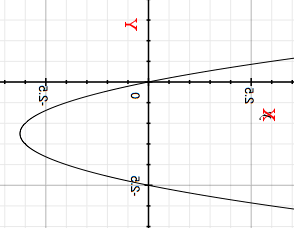SEARCH HOMEMath Central Quandaries & QueriesQuestion from Ashini, a student: The inverse function for f(x)=2x^2+5xHi Ashini,

The first thing I would is plot $y = 2x^2 + 5x$ as I did in my response to a similar question. I then relabeled the X-axis as Y and the Y-axis as X and put the graph in standard position with the new X-axis horizontal and the Y-axis vertical I getThus you can see that the inverse is composed of two functions, one that is the top half of the parabola and the other the bottom half of the parabola.

To find an expression for these functions use the technique suggested by RD.

Write back if you need further assistance,
PennyMath Central is supported by the University of Regina and the Imperial Oil Foundation.